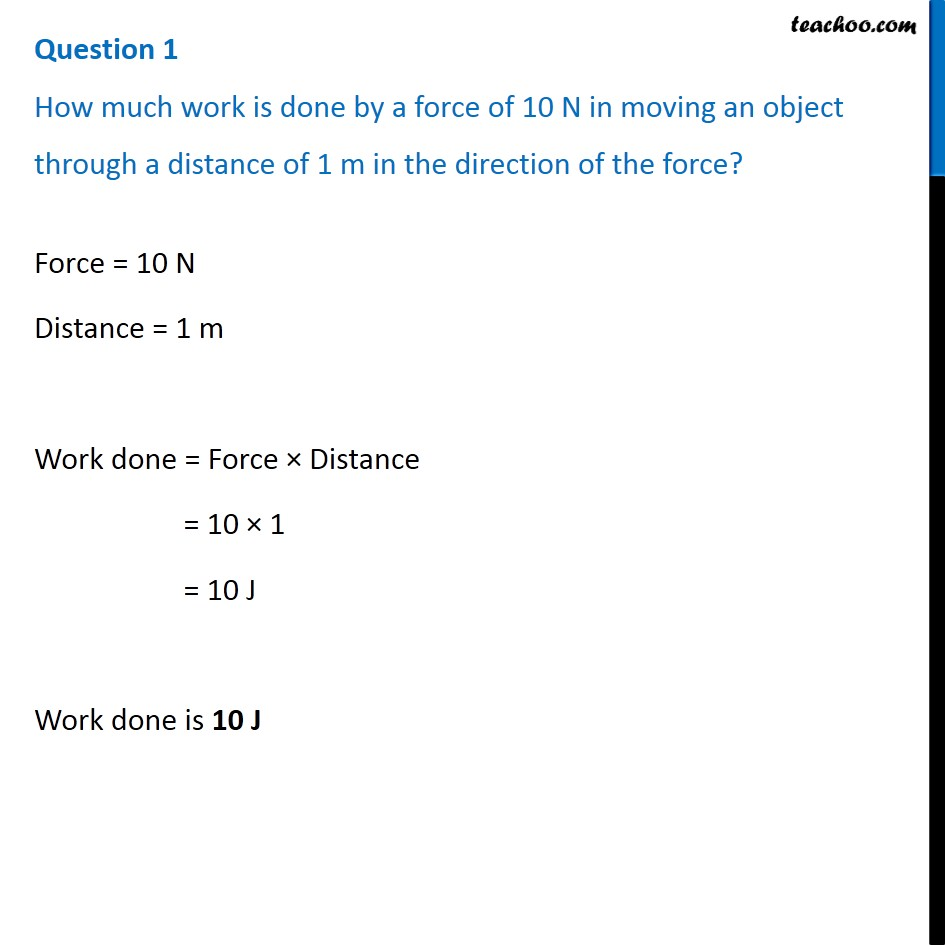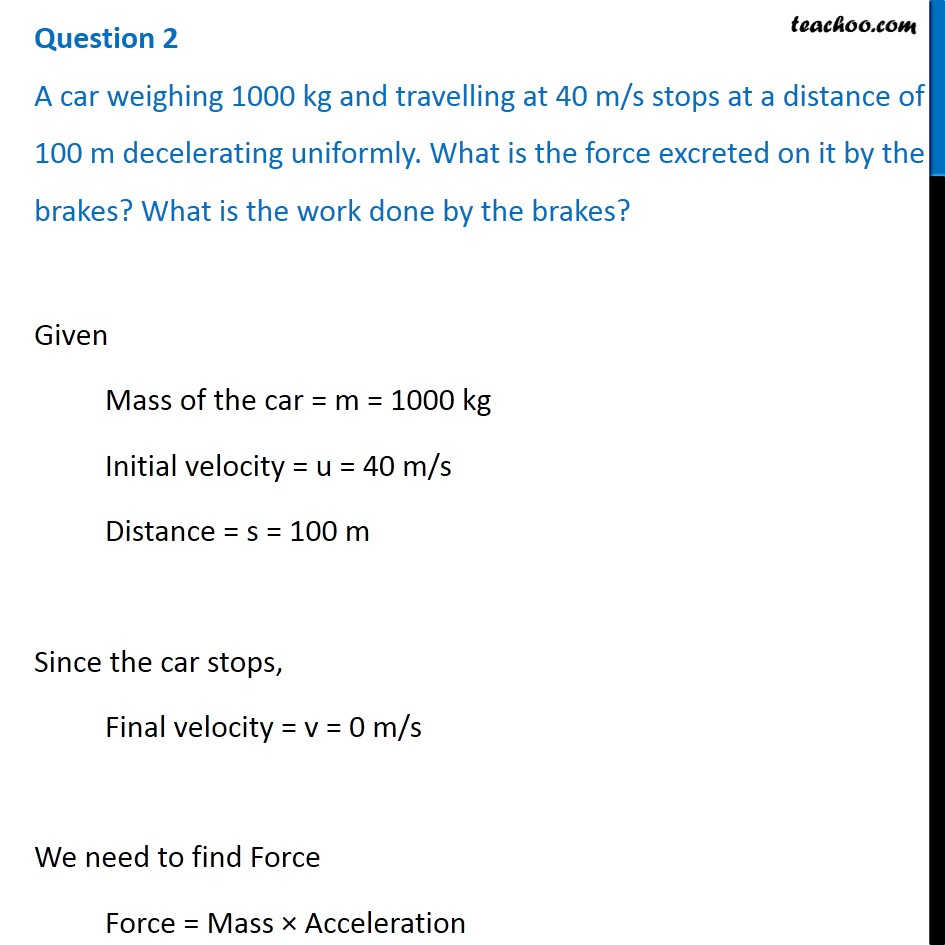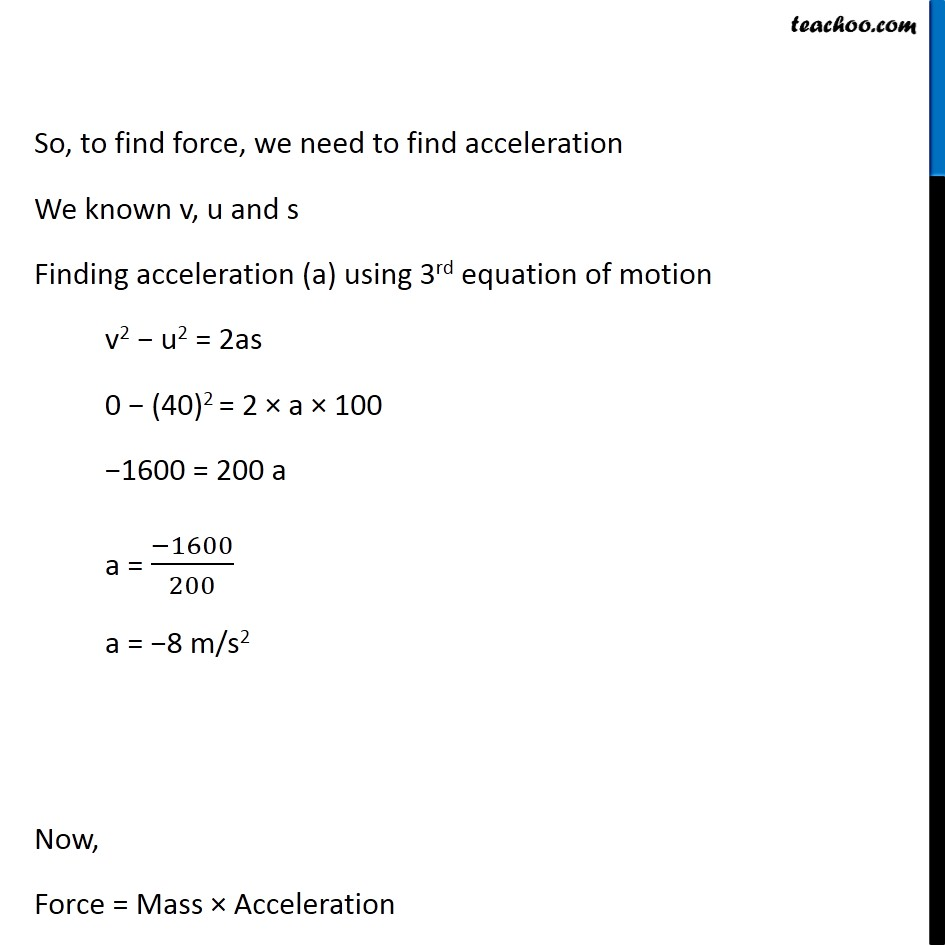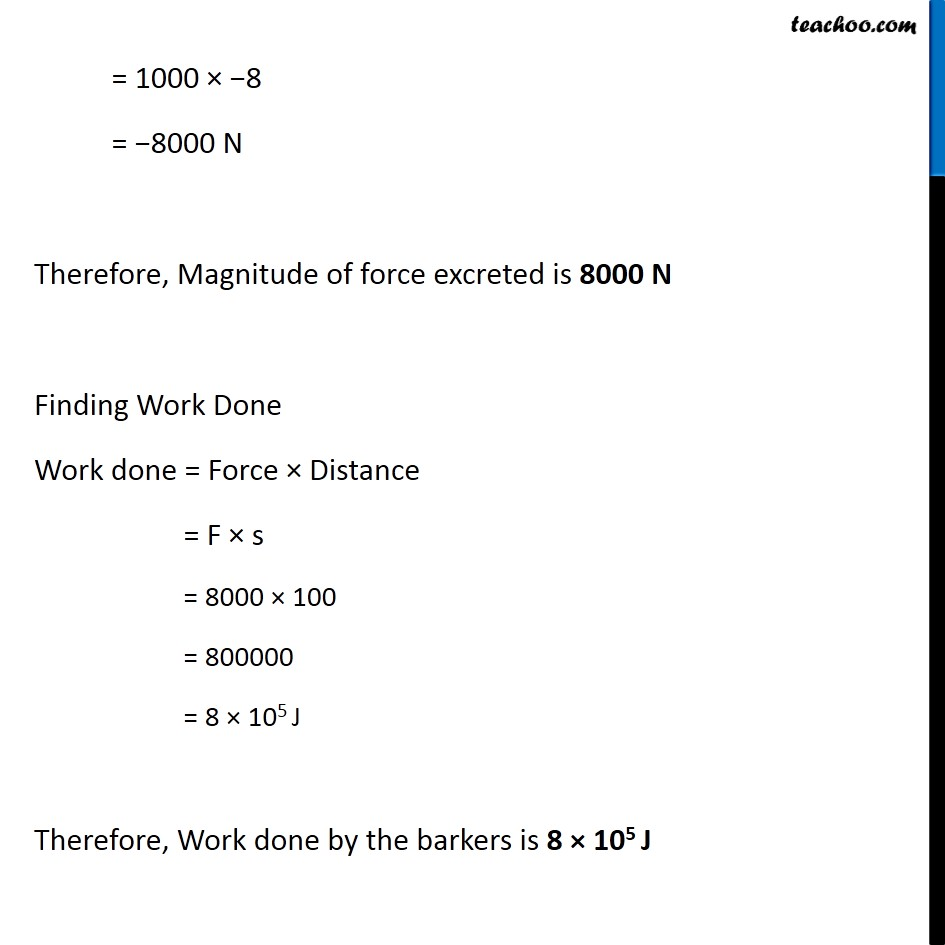Concepts

Class 9
Chapter 11 Class 9 - Work and Energy

In general language, any thing we do is work

Example

Reading, Writing, Teaching, Running, Working on Computer

## What is definition of work in Physics

Work is done when a force produces motion.

## Important Points

### Work Requires some force

(Example - To run, we require muscular force)

### Some motion shall be produced

Some distance shall be travelled in direction of force

Example - if we push a wall but wall does not move, so it is not work.

But if we push a car and it moves in direction of force, it is work.

## What is formula for calculating Work

Work = Force × Distance moved in particular direction

We can say that

Work = Force × Displacement

W = F × s

## What is SI Unit of Work

Work is measured in Joule

1 Joule = 1 Newton Meter

1 J = 1Nm

Explantion

Since Force is measured in Newton (N)

Distance/Displacement is measured in Meter(m)

Work done by 1 Newton of Force to produce displacement of 1 meter = 1 Joule

Work = Force × Distance

= 1 N × 1 m

= 1 Joule

## QuestionsQ 1 Page 148 - A force of 7 N acts on an object. The displacement is, say 8 m, in the direction of the force (Fig. 11.3). Let us take it that the force acts on the object through the displacement. What is the work done in this case?

Q 1 Page 149 - When do we say that work is done?

Q 2 Page 149 - Write an expression for the work done when a force is acting on an object in the direction of its displacement

Q 3 Page 149 - Define 1 J of work.

Q 4 Page 149 - A pair of bullocks exerts a force of 140 N on a plough. The field being ploughed is 15 m long. How much work is done in ploughing the length of the field?

Example 11.1 - A force of 5 N is acting on an object. The object is displaced through 2 m in the direction of the force (Fig. 11.2).

Example 11.2 - A porter lifts a luggage of 15 kg from the ground and puts it on his head 1.5 m above the ground. Calculate the work done by him on the luggage.

NCERT Question 1 - Look at the activities listed below. Reason out whether or not work is done in the light of your understanding of the term ‘work’.

NCERT Question 2 - An object thrown at a certain angle to the ground moves in a curved path and falls back to the ground. The initial and the final points of the path of the object lie on the same horizontal line. What is the work done by the force of gravity on the object?

Learn in your speed, with individual attention - Teachoo Maths 1-on-1 Class

### Transcript

Question 1 How much work is done by a force of 10 N in moving an object through a distance of 1 m in the direction of the force? Force = 10 N Distance = 1 m Work done = Force × Distance = 10 × 1 = 10 J Work done is 10 J Question 2 A car weighing 1000 kg and travelling at 40 m/s stops at a distance of 100 m decelerating uniformly. What is the force excreted on it by the brakes? What is the work done by the brakes? Given Mass of the car = m = 1000 kg Initial velocity = u = 40 m/s Distance = s = 100 m Since the car stops, Final velocity = v = 0 m/s We need to find Force Force = Mass × Acceleration So, to find force, we need to find acceleration We known v, u and s Finding acceleration (a) using 3rd equation of motion v2 − u2 = 2as 0 − (40)2 = 2 × a × 100 −1600 = 200 a a = (−1600)/200 a = −8 m/s2 Now, Force = Mass × Acceleration = 1000 × −8 = −8000 N Therefore, Magnitude of force excreted is 8000 N Finding Work Done Work done = Force × Distance = F × s = 8000 × 100 = 800000 = 8 × 105 J Therefore, Work done by the barkers is 8 × 105 J